International
Tables for
Crystallography
Volume F
Crystallography of biological macromolecules
Edited by M. G. Rossmann and E. Arnold

International Tables for Crystallography (2006). Vol. F, ch. 11.4, pp. 226-227   | 1 | 2 |

Section 11.4.2. Diffraction from a perfect crystal lattice

Z. Otwinowskia* and W. Minorb

aUT Southwestern Medical Center at Dallas, 5323 Harry Hines Boulevard, Dallas, TX 75390-9038, USA, and bDepartment of Molecular Physiology and Biological Physics, University of Virginia, 1300 Jefferson Park Avenue, Charlottesville, VA 22908, USA
Correspondence e-mail:  zbyszek@mix.swmed.edu

11.4.2. Diffraction from a perfect crystal lattice

| top | pdf |

X-ray photons can scatter from individual electrons by inelastic and incoherent processes. The coherent scattering by the whole crystal is called diffraction.1 Energy conservation, when expressed in photon momentum vectors, is equivalent to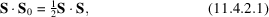where S is the diffraction vector, defined as the change of photon momentum in the scattering process, andis the vector which has beam direction and length. Diffraction from a perfect crystal lattice occurs when diffraction from all repeating crystal elements is in phase, which can be stated in vector algebra as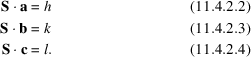In shorter notation, these may be written as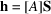, which is equivalent to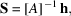whereare the real-space crystal periodicity vectors,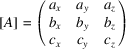and h, k, l are the integer Miller indices. Often, the orientation matrix is defined in reciprocal space as the inverse of [A].

The condition for crystal diffraction with Miller indices h, k, l is the existence of a (unique) vector S which is a solution to equations (11.4.2.1)–(11.4.2.4). Equation (11.4.2.1) states the diffraction condition for vector S. Mathematically speaking, the space of the solutions to equations (11.4.2.2)–(11.4.2.4) is called reciprocal space, and vector S belongs to this space. However, the following presentation does not depend on the properties of reciprocal space. The laboratory coordinate system used has its origin at the position of the crystal. A diffraction peak at the detector position in three-dimensional laboratory space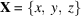corresponds to vector S: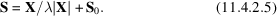Rotation of the crystal around the goniostat axes can be described by vectorsin equations (11.4.2.2)–(11.4.2.4) as a function of the goniostat angles, and vectors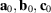represent the crystal orientation at the zero position of the goniostat. These rotations are described by Bricogne (1987):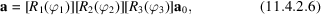where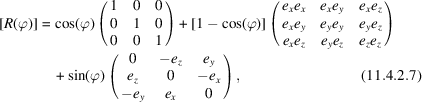where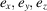represent the direction cosines of a rotation axis. To complete the description of diffraction geometry, we need a function X(p, q), describing the position in experimental space of each pixel with integer coordinates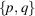. This function is detector-specific and describes the detector geometry and distortion. For a planar detector,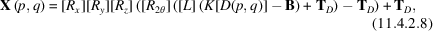where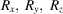represent the detector misorientation,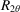represents rotation around the 2θ (swing) axis,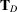is the detector translation from the crystal, operation L represents the axis naming/direction convention used by the detector manufacturer (eight possibilities), K is an operation scaling pixels to millimetres, D is a detector distortion function and B represents the beam position on the detector surface.

Equations (11.4.2.1)–(11.4.2.8) fully describe the existence and position of the diffraction peaks, which is all that is needed for the autoindexing procedure.

References

Bricogne, G. (1987). The EEC cooperative programming workshop on position-sensitive detector software. In Proceedings of the Daresbury study weekend at Daresbury Laboratory, 23–24 January, edited by J. R. Helliwell, P. A. Machin and M. Z. Papiz, pp. 120–146. Warrington: Daresbury Laboratory.Google Scholar